Question

# I need to know if I calculated the acetate buffer correctly & how to calculate the...

I need to know if I calculated the acetate buffer correctly & how to calculate the change in pH when HCl is added...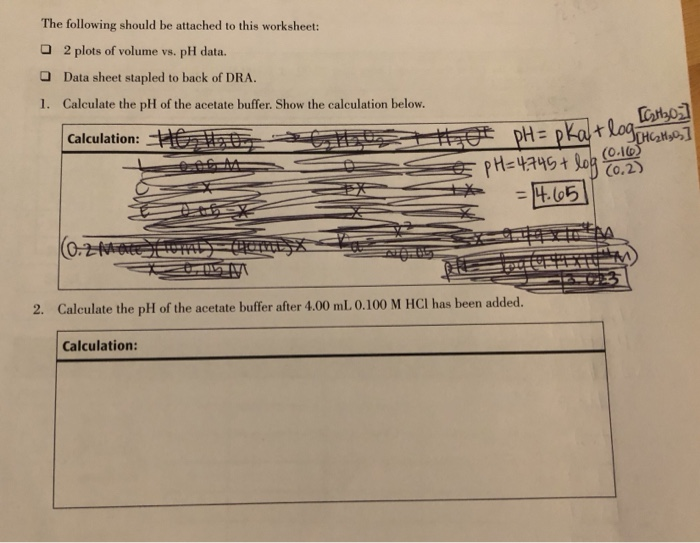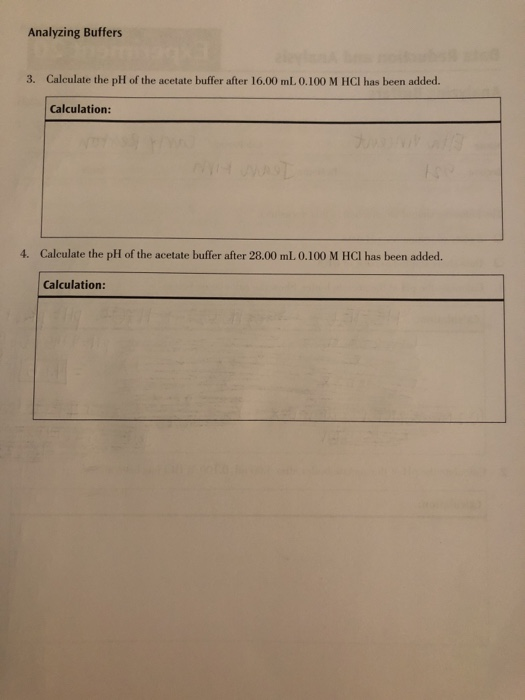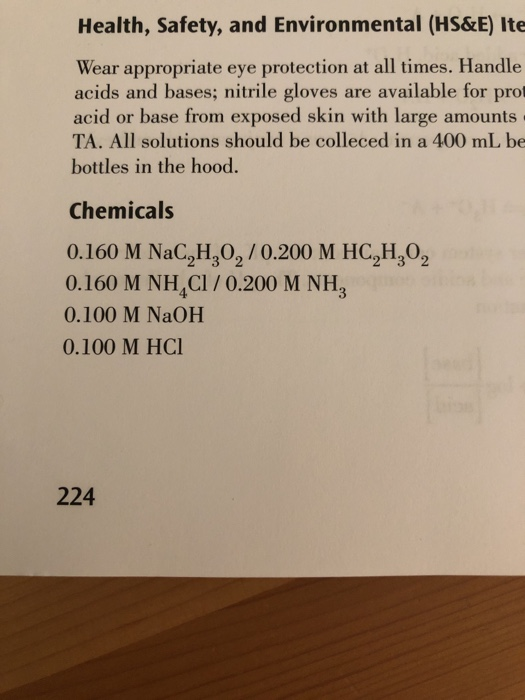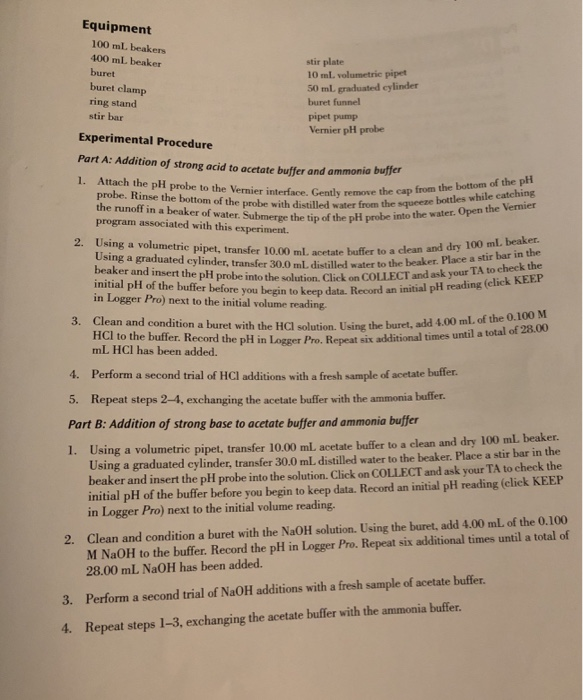The following should be attached to this worksheet: 2 plots of volume vs. pH data. Data sheet stapled to back of DRA. 1. Calculate the pH of the acetate buffer. Show the calculation below. Calculation: Ho t t 419 [Cathoz ot pH= pkatlog HGH sos] Œ pH=4.745 + log NAH ** = 4.65 (0.2 m m 2. Calculate the pH of the acetate buffer after 4.00 mL 0.100 M HCl has been added. Calculation:
Analyzing Buffers 3. Calculate the pH of the acetate buffer after 16.00 mL. 0.100 M HCl has been added Calculation: 4. Calculate the pH of the acetate buffer after 28.00 mL 0.100 M HCl has been added. Calculation:
Health, Safety, and Environmental (HS&E) Ite Wear appropriate eye protection at all times. Handle acids and bases; nitrile gloves are available for prot acid or base from exposed skin with large amounts TA. All solutions should be colleced in a 400 ml be bottles in the hood. Chemicals 0.160 M NaC,H,O,/0.200 M HC,H,O, 0.160 M NH C1 / 0.200 M NH, 0.100 M NaOH 0.100 M HCl 224

(2 ) In 10 mL buffer solution which is diluted by 30 mL of distilled water 0.1 M , 4 mL HCl is added.

since acetate is basic part of buffer solution it will react with added HCl solution.calculation is as following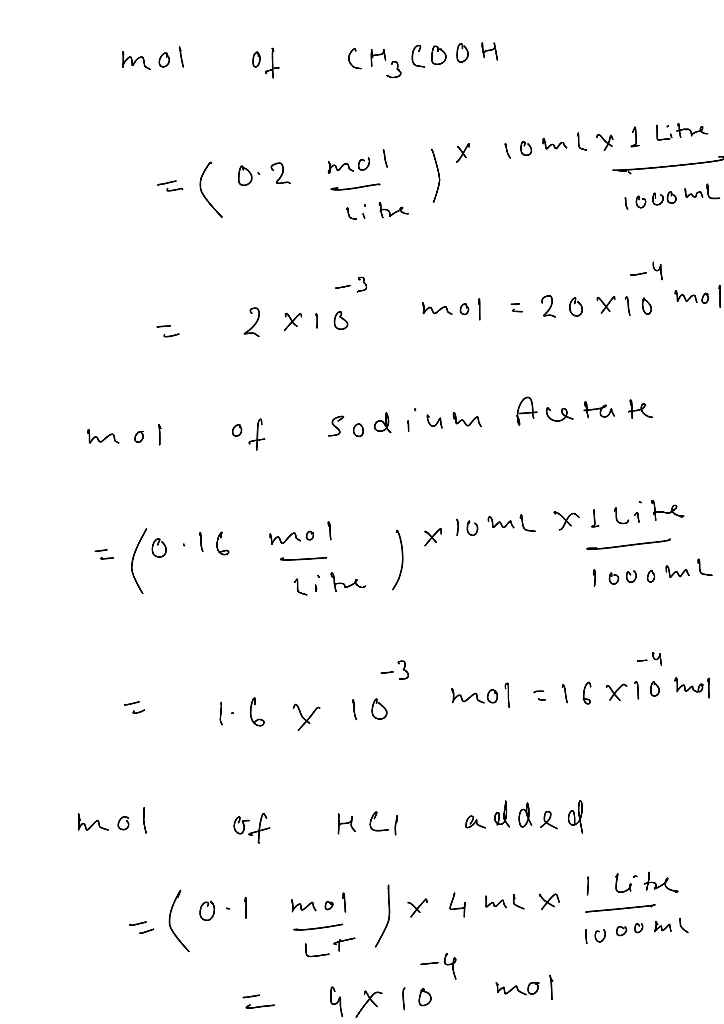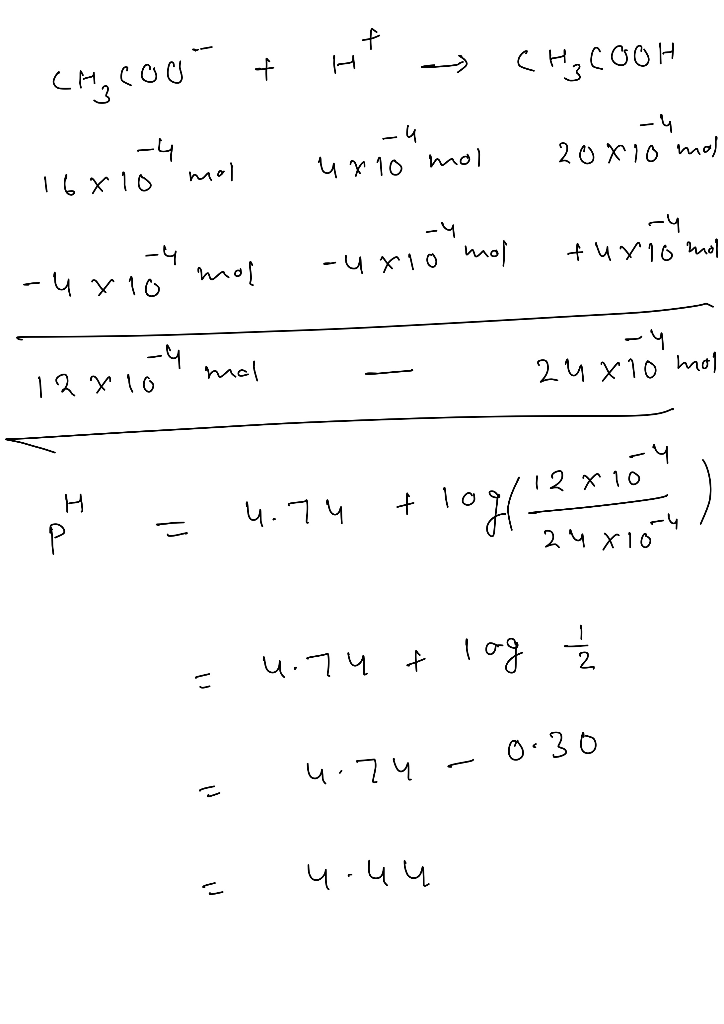#### Earn Coins

Coins can be redeemed for fabulous gifts.

Similar Homework Help Questions
• ### based on instructions please help me fill out chart Use appropriate safety precautions for working with...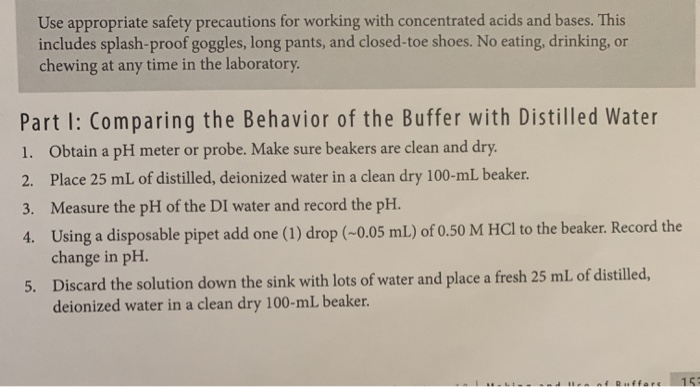based on instructions please help me fill out chart Use appropriate safety precautions for working with concentrated acids and bases. This includes splash-proof goggles, long pants, and closed-toe shoes. No eating, drinking, or chewing at any time in the laboratory. Part I: Comparing the Behavior of the Buffer with Distilled Water 1. Obtain a pH meter or probe. Make sure beakers are clean and dry. 2. Place 25 mL of distilled, deionized water in a clean dry 100-ml beaker. 3....

• ### 1. Calculate the pH of the acetate buffer, mmol. HA, mmol. A-, and mmol. H3O+ after...

1. Calculate the pH of the acetate buffer, mmol. HA, mmol. A-, and mmol. H3O+ after 4mL, 8mL, 12mL, 16mL, 20mL, 24mL, and 28mL of 0.100M HCl is added to the acetate buffer. 2. Calculate the pH of the acetate buffer, mmol. HA, mmol. A-, and mmol. H3O+ after 4mL, 8mL, 12mL, 16mL, 20mL, 24mL, and 28mL of 0.100M NaOH is added to the acetate buffer. 3. Calculate the pH of the ammonia buffer, mmol. HA, mmol. A-, and mmol....

• ### ALL THE INFO YOU NEED IS BELOW average volume of NaOH: 15.7 mL or 0.0157 L...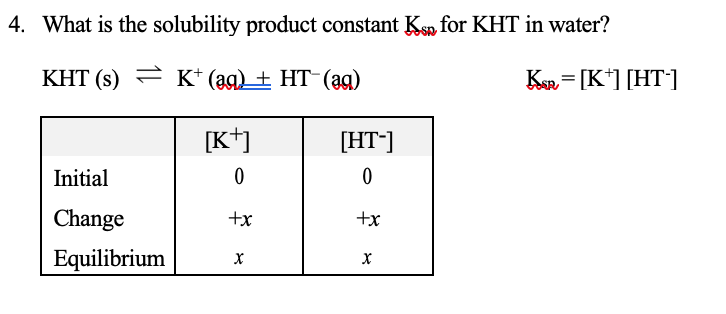ALL THE INFO YOU NEED IS BELOW average volume of NaOH: 15.7 mL or 0.0157 L average number of moles of NaOH added: 6.28*10-4 moles molar concentration of [HT] in saturated solution(HT– acts as a monoprotic acid, reacting with NaOH in a 1:1 ratio (Volume of KHT measured using 25.00 mL volumetric pipet.)): 0.2512 M Analysis of KHT in water 1. Weigh out 0.8 grams of potassium hydrogen tartrate (KHT) on weighing paper. Add the KHT to a clean dry...

• ### how to calculate the buffer capacity and how can i solve the properties of buffer 2...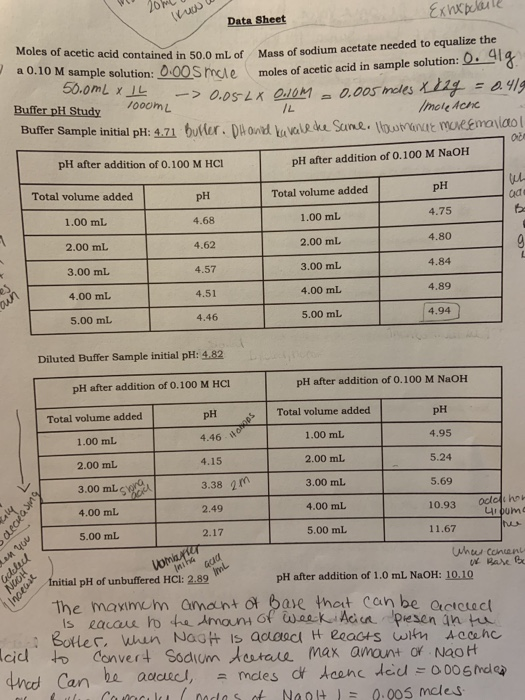how to calculate the buffer capacity and how can i solve the properties of buffer 2 solution. i already some calculations put im not sure if I'm correct КИХр. Data Sheet Moles of acetic acid contained in 50.0 mL of a 0.10 M sample solution: 0.00 Smule 50.0mL XL -> 0.05-24 0.1om Buffer pH Study 1000mL IL Buffer Sample initial pH: 4.71 buller. DH and kavalehe Sume. How mane marremalol pH after addition of 0.100 M HCI Mass of sodium...

• ### Preparation of Buffer Solutions (2 pts each) Mass of sodium acetate in acetate buffer 1.9821 3.00...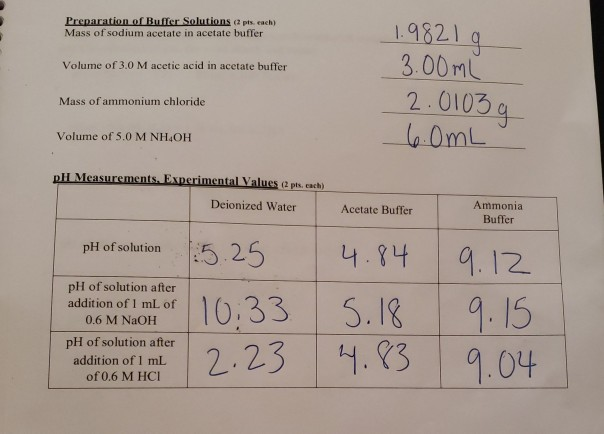Preparation of Buffer Solutions (2 pts each) Mass of sodium acetate in acetate buffer 1.9821 3.00m 2.0103 Volume of 3.0 M acetic acid in acetate buffer Mass of ammonium chloride Volume of 5.0 M NH4OH pH Mcasurements.Experimental Valucs epts. eachi Deionized Water Ammonia Buffer Acetate Buffer 5 25 4.4 9.12 .33 5.1 23.3 9.04 pH of solution płł of solution after addition of I mL of 0.6 M NaOH pH of solution after addition of 1 mL of 0.6 M...

• ### Prepare dilute acid and base solutions and buffered acid and base solutions to observe how buffering...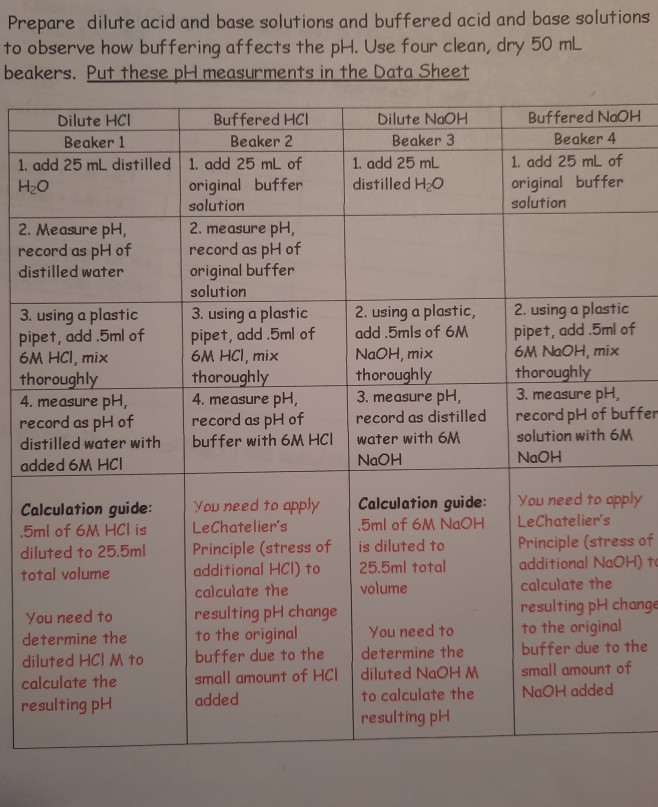Prepare dilute acid and base solutions and buffered acid and base solutions to observe how buffering affects the pH. Use four clean, dry 50 ml beakers. Put these pH measurments in the Data Sheet Dilute NaOH Beaker 3 1. add 25 mL distilled H2O Buffered NaOH Beaker 4 1. add 25 mL of original buffer solution Dilute HCI Buffered HCI Beaker 1 Beaker 2 1. add 25 mL distilled 1. add 25 mL of H2O original buffer solution 2. Measure...

• ### For the buffer we prepared in the procedure, when the buffering effect fail? (Hint: This is...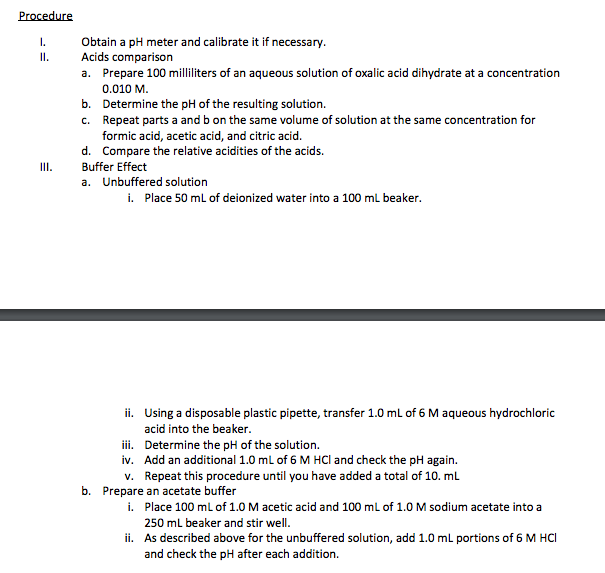For the buffer we prepared in the procedure, when the buffering effect fail? (Hint: This is equivalent to asking when will we exceed the buffer's capacity?) Procedure I. Obtain a pH meter and calibrate it if necessary ll. Acids comparison a. Prepare 100 milliliters of an aqueous solution of oxalic acid dihydrate at a concentration 0.010 M b. Determine the pH of the resulting solution. c. Repeat parts a and b on the same volume of solution atthe same concentration...

• ### According to the Henderson-Hasselbalch equation, how many grams of sodium acetate will you need to add to 125 mL of 0.100 M acetic acid to make a pH=4.74 buffer

a. According to the Henderson-Hasselbalch equation, how many grams of sodium acetate will you need to add to 125 mL of 0.100 M acetic acid to make a pH=4.74 buffer? b. What is the actual pH of the resulting buffer when you add that many grams of sodium acetate to 125 mL of 0.100 M acetic acid? c. If I want to make the buffer by adding 0.100 M NaOH instead of sodium acetate, how many milliliters of NaOH are...

• ### Please help with the prelab questions! thank you!!!! Especially 2 and 3! Pre-Lab Questions 1. Calculate...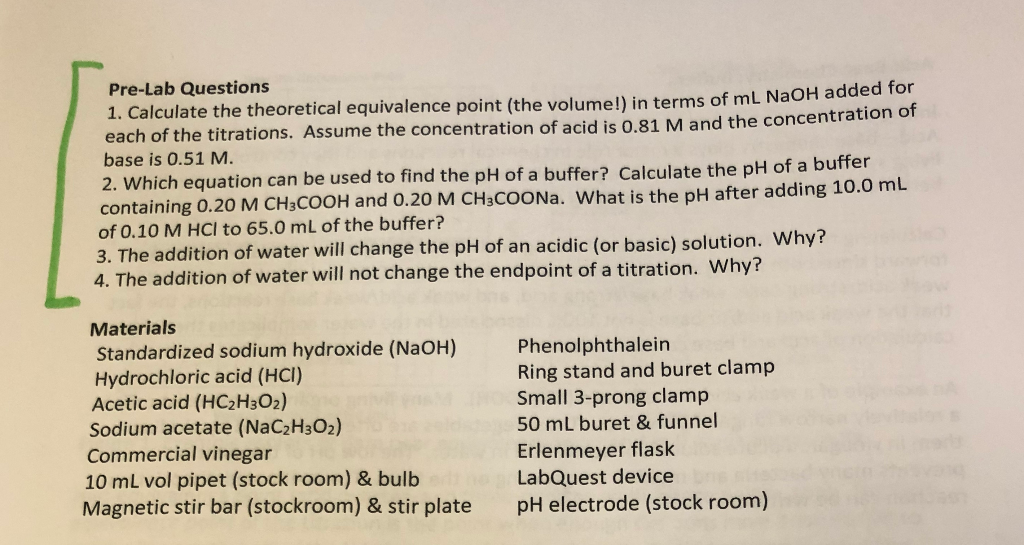Please help with the prelab questions! thank you!!!! Especially 2 and 3! Pre-Lab Questions 1. Calculate the theoretical equivalence point (the volume!) in terms of ml NaOH adde each of the titrations. Assume the concentration of acid is 0.81 M and the concentration of base is 0.51 M. 2. Which equation can be used to find the pH of a buffer? Calculate the pH of a buffer containing 0.20 M CH3COOH and 0.20 M CH3COONa. What is the pH after...

• ### EXPERIMENT Provided Materials and Equipment: General glassware found in the lab or in your drawer/locker 60...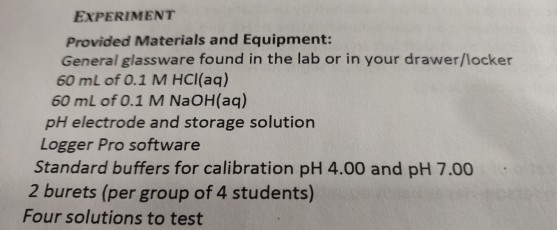EXPERIMENT Provided Materials and Equipment: General glassware found in the lab or in your drawer/locker 60 mL of 0.1 M HCl(aq) 60 mL of 0.1 M NaOH(aq) pH electrode and storage solution Logger Pro software Standard buffers for calibration pH 4.00 and pH 7.00 2 burets (per group of 4 students) Four solutions to test For the acid: Pour 60 mL of HCL in a buret using a funnel. Prepare 25 mL of each solution in a separate beaker (water,...